# Matrix | What is Matrix?## Matrix | What is Matrix?

A matrix is a rectangular array of numbers arranged in horizontal and vertical lines and enclosed between round or square brackets. Horizontal lines are called rows and vertical lines are called columns of a matrix.

********************

10 Math Problems officially announces the release of Quick Math Solver, an Android App on the Google Play Store for students around the world.

********************

Some examples of the matrix are given below:Each member or number in the array is called an element or an entry of the matrix. An element appearing in the ith row and jth column of a marix is called its (i, j)th element or the (i, j)th entry.

### Order of a Matrix

The order or the size of the matrix is given by the number of rows followed by the number of columns. If a matrix contains m rows and n columns, then it is of order m × n, read as m by n.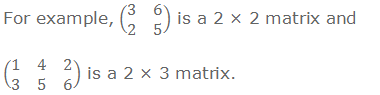### Matrix Notation

The matrices are usually denoted by capital letters such as A, B, C, ………etc. The elements are denoted by the corresponding small letters along with two suffixes. The first suffix indicates the number of row and latter one indicates the number of column in which the element appears.

aij is the element of a matrix A in the ith row and jth column.

a23 is the element of a matrix A in the 2nd row and 3rd column.

Thus a matrix of order m × n may be written as

A = (aij)m × n

If A is a 3 × 3 matrix, then it may be written as### Types of Matrices

1.    Row Matrix: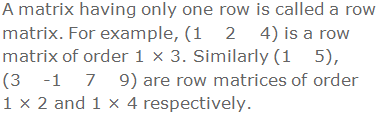2.    Column Matrix: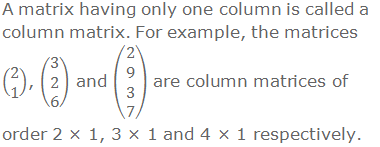3.    Square Matrix:4.    Rectangular Matrix:5.    Zero Matrix or Null Matrix:6.    Diagonal Matrix: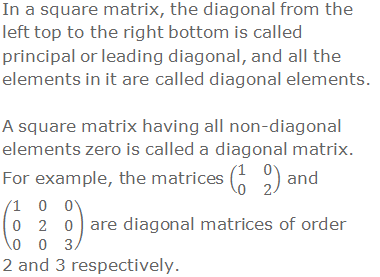7.    Scalar Matrix:8.    Unit Matrix or Identity Matrix: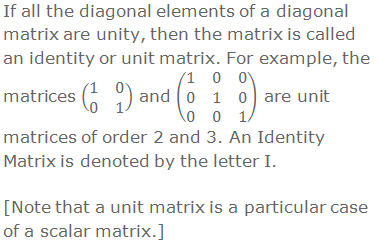### Equal Matrices

Two matrices A and B are said to be equal matrices if A and B are of same order i.e. number of rows in A = number of rows in B and number of columns in A = number of columns in B, and their corresponding elements are equal i.e. the entries of A and B in the same position are equal. Otherwise, the matrices are said to be unequal.

If A and B are equal matrices, then we write A = B. Otherwise, we write A ≠ B.

For example:### Worked Out Examples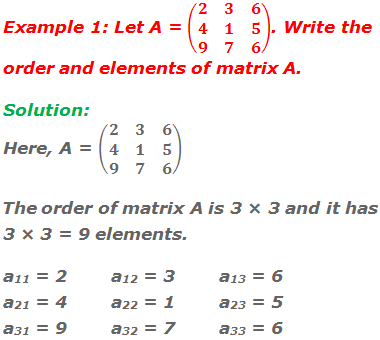Example 2: If a matrix has 6 elements, what are the possible orders it can have?

Solution:

If a matrix has 6 elements, then it can have any one of the following orders:

1 × 6, 6 × 1, 2 × 3 or 3 × 2.

Example 3: Construct a 2 × 2 matrix whose elements aij are given by aij = i + j.Example 4: Construct a 2 × 2 matrix A whose element aij are given by aij = 3i + 2j.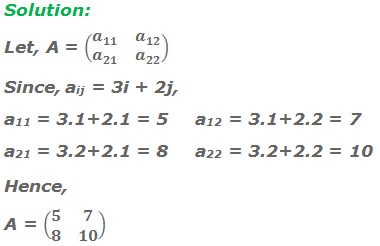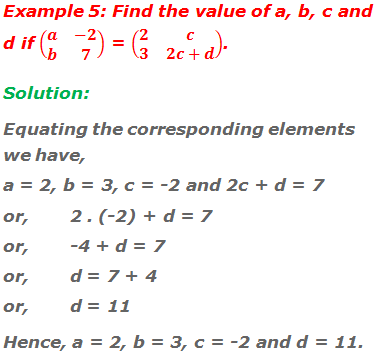#### Do you have any questions regarding the matrix?

You can ask your questions or problems here, in the comment section below.